# Texas Go Math Grade 5 Lesson 10.1 Answer Key Number Patterns

Refer to our Texas Go Math Grade 5 Answer Key Pdf to score good marks in the exams. Test yourself by practicing the problems from Texas Go Math Grade 5 Lesson 10.1 Answer Key Number Patterns.

## Texas Go Math Grade 5 Lesson 10.1 Answer Key Number Patterns

Unlock the Problem

Luisa is making flan. For every egg, she can make three flans. The rule f= 3e describes the relationship between the number of flans, f and the number of eggs, e. How many flans can Luisa make if she uses 5 eggs?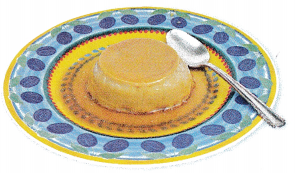You can use an input/output table or a list to show a pattern. When a pattern is shown in a table, it shows the relationship between the inputs and outputs. When a pattern is shown in a list, it only shows the outputs in order.

Complete the input/output table. Replace e in the equation f= 3e with the value shown in the table to find the value of the output f.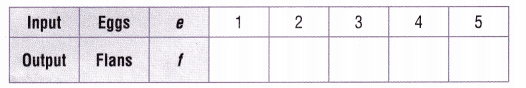The pattern for the number of flans is 3, 6, ______, ______, ______.
The fifth number in the pattern is ______.
So, Luisa can make ______ flans.The pattern for the number of flans is 3, 6, 9, 12, 15,
The fifth number in the pattern is 15,
Luisa can make 15 flans if she uses 5 eggs,

Explanation:
Given Luisa is making flan. For every egg, she can make three flans.
The rule f= 3e describes the relationship between the number of flans,
f and the number of eggs, e
The pattern for the number of flans is shown in
above the input/output table is e = 1, f = 3 X 1 = 3 flans
if e = 2, f =  3 X 2 = 6 flans,
if e = 3, f = 3 X 3 = 9  flans,
if e = 4, f = 3 X 4 = 12 flans,
if e = 5, f = 3 X 5 = 15 flans,
therefore the fifth number in the pattern is 15,
So Luisa can make 15 flans if she uses 5 eggs.

Math Talk
Mathematical Processes

Explain how you can use the pattern to find how many flans can be made using 9 eggs.
Number of flans can be made using 9 eggs is 27 flans,

Explanation:
We use the rule f= 3e which describes the relationship between
the number of flans f and the number of eggs e
The number of flans can be made using 9 eggs is
f= 3 X 9 = 27 flans, therefore number of flans can be made
using 9 eggs is 27 flans.

What if the Luisa changed her recipe to make 4 flans with every egg? How would the pattern change?
The pattern changes to f = 4e,

Explanation:
Before Luisa is making flan. For every egg, she can make three flans.
The rule f= 3e describes the relationship between
the number of flans f and the number of eggs e,
now Luisa changed her recipe to make 4 flans with every egg instead of 3,
so very simple the pattern changes to f = 4e where f is the number of flans
and e is the number of eggs respectively.

Example
Find the pattern.
The rule for the number of circles in a figure is c = f + 2
where c is the number of circles and f is the figure number.
How many circles will be in Figure 8?The pattern for the number of circles is 3, 4, ______, ______, ______, ______, ______, ______.
The eighth number in the pattern is ______.
So, there are ______ circles in Figure 8.The pattern for the number of circles is 3, 4, 5, 6, 7, 8, 9, 10,
The eighth number in the pattern is 10,
So, there will be 10 circles in Figure 8,

Explanation:
Given the rule for the number of circles in a figure is c = f + 2
where c is the number of circles and f is the figure number,
Completed the the input/output table as shown above
The pattern for the number of circles is
if f = 1, c = 1 + 2 = 3,
if f = 2, c = 2 + 2 = 4,
if f = 3, c = 3 + 2 = 5,
if f = 4, c = 4 + 2 = 6,
if f = 5, c = 5 + 2 = 7,
if f = 6, c = 6 + 2 = 8,
if f = 7, c = 7 + 2 = 9,
if f =8, c = 8 + 2 = 10,
therefore the eighth number in the pattern is 10,
So, there will be 10 circles in Figure 8.

Share and Show

Use the rule to complete the table.

Question 1.
Rule: g = n + 6.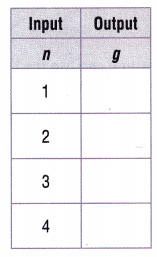Explanation:
Used the rule g = n + 6 as
if n = 1, g= 1 + 6 = 7,
if n = 2, g = 2 + 6 = 8,
if n = 3, g  = 3 + 6 = 9,
if n = 4, g = 4 + 6 = 10 and completed the table as shown above.

Question 2.
Rule: p = r × 12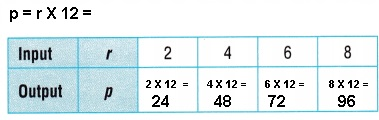Explanation:
Used the rule p = r X 12 as
if r = 2, p = 2 X 12 = 24,
if r = 4, p = 4 X 12 = 48,
if r = 6, p = 6 X 12 = 72,
if r = 8, p = 8 X 12 = 96 and completed the table as shown above.

Problem Solving

Practice: Copy and Solve Use the rule to make an input/output table.
Include four input/output pairs in your table.

Question 3.
For input x and output y, the rule is y = x + 4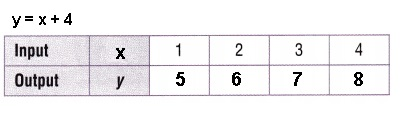Explanation:
Used the rule y = x + 4 as
if  x = 1, y = 1 + 4 = 5,
if  x = 2, y = 2 + 4 = 6,
if  x = 3, y = 3 + 4 = 7,
if  x = 4, y = 4 + 4 = 8 and completed the table as shown above.

Question 4.
For input s and output a, the rule is a = 11 sExplanation:
Used the rule a = 11s as
if  s = 1, a = 11 X 1 = 11,
if  s = 2, a = 11 X 2 = 22,
if  s = 3, a = 11 X 3 = 33,
if  s = 4, a = 11 X 4 = 44 and completed the table as shown above.

Question 5.
For input u and output v, the rule is v = 7uExplanation:
Used the rule v = 7u as
if  u = 1, v = 7 X 1 = 7,
if  u = 2, v = 7 X 2 = 14,
if  u = 3, v = 7 X 3 = 21,
if  u = 4, v = 7 X 4 = 28 and completed the table as shown above.
Question 6.
For input m and output n, the rule is n = m × 10Explanation:
Used the rule n = m X 10 as
if  m = 1, n = 1 X 10 = 10,
if  m = 2, n = 2 X 10 = 20,
if  m = 3, n = 3 X 10 = 30,
if  m = 4, n = 4 X 10 = 40 and completed the table as shown above.

Question 7.
H.O.T. Explain how you can use the formula for the perimeter of a square,
P = 4s, to generate a pattern. Use the pattern to find the perimeter of a
square with sides that are 5 cm long.
The perimeter of a square with sides that are 5 cm long is
p = 20 cm,

Explanation:
Given and we know to use the formula for the perimeter of a square,
P = 4s, to generate a pattern. So using the pattern in finding
the perimeter of a square with sides that are 5 cm long is simple
here s is 5 cm, therefore p = 4 X 5 cm = 20 cm.

Problem Solving

Question 8.
Max makes origami paper cranes for a mobile.
He can make 5 cranes with each sheet of paper.
Complete the input/output table to show the
number of cranes Max can make with 6 sheets of paper.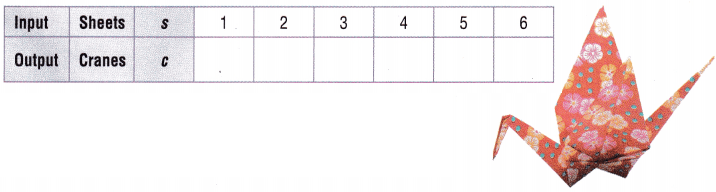number of cranes Max can make with 6 sheets of paper are 30,

Explanation:
Given Max makes origami paper cranes for a mobile.
He can make 5 cranes with each sheet of paper.
So pattern is cranes  =  5 X sheet, So if
s = 1 , c = 5 X 1 = 5,
if s = 2, c = 5 X 2 = 10,
if s = 3, c = 5 X 3 = 15,
if s= 4, c = 5 X 4 = 20,
if s = 5, c = 5 X 5 = 25 and
if s = 6, c= 6 X 5 = 30,
Completed the input/output table to show the number of cranes
Max can make with 6 sheets of paper are 30 above.

Question 9.
H.O.T. Multi-Step Max decides to make paper owls.
He can make 4 paper owls from each sheet of paper.
Complete and use the input/output table to find how many sheets of
paper Max would use to make 24 paper owls.Max can use 6 sheets of paper to make 24 paper owls,

Explanation:
Given Max decides to make paper owls.
He can make 4 paper owls from each sheet of paper.
So pattern is owls  =  4 X sheet, So if
if s = 1, o = 4 X 1 = 4,
if s = 2, o = 4 X 2 = 8,
if s = 3, o = 4 X 3 = 12,
if s= 4, o = 4 X 4 = 16,
if s = 5, o = 5 X 4 = 20 and
if s = 6, o = 6 X 4 = 24,
Completed the input/output table to show the number of owls above
so as per table and pattern Max can use 6 sheets of paper
to make 24 paper owls.

Question 10.
Apply Matilda is reading a map of the route to her grandfather’s house in another state.
The equation m = 25i describes the relationship between i,
the number of inches on the map, and m, the number of actual miles.
How many miles are represented by 12 inches?
(A) 200 miles
(B) 600 miles
(C) 400 miles
(D) 300 miles
The number of miles that are represented by 12 inches are 300 miles
matches with (D),

Explanation:
Given Matilda is reading a map of the route to her grandfather’s house in another state.
The equation m = 25i describes the relationship between i,
the number of inches on the map and m the number of actual miles.
So number of miles that are represented by 12 inches are
applying in m = 25i we get  25 X 12 = 300 miles which is (D).

Question 11.
To find the total cost of a field trip, use the equation c = 4s,
where s is the number of students and c is the cost of the field trip.
How much will it cost for 14 students to attend the field trip?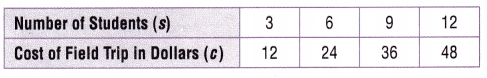(A) $15 (B)$17
(C) $56 (D)$60
It will cost for 14 students to attend the field trip is $56, So (C), Explanation: Given to find the total cost of a field trip, using the equation c = 4s, where s is the number of students and c is the cost of the field trip. So it cost for 14 students to attend the field trip is c = 4 X 14 =$56
which matches with (C).

Question 12.
Multi-Step Robin places 3 roses and 2 daffodils in each vase.
How many flowers will she need if she has 6 vases?
(A) 30
(B) 12
(C) 18
(D) 5
30 flowers Robin needs is she has 6 vases,
match is (A),

Explanation:
Given Robin places 3 roses and 2 daffodils in each vase means
in each vase she is keeping 1 vase = 3 + 2 = 5 flowers(5 f)
so 1 vase  =  5f here v is vase and f  is flowers, therefore 6 vases will have
6 X 5 = 30 flowers which matches with (A).

Texas Test Prep

Question 13.
Sergei uses the pattern rule y = 4 + b to generate the first three outputs in the pattern.
Which of the following is the fifth output in the pattern?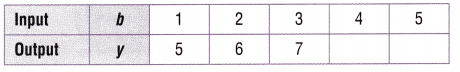(A) 5
(B) 9
(C) 8
(D) 10
9, matches with (B),

Explanation:
Given Sergei uses the pattern rule y = 4 + b to generate
the first three outputs in the pattern. The following is the fifth
output in the pattern is y = 4 + 5 = 9 which matches with (B).

### Texas Go Math Grade 5 Lesson 10.1 Homework and Practice Answer Key

Use the rule to make an input/output table. Include four input/output pairs in your table.

Question 1.
Rule: The output is a + 5.Explanation:
Given the rule output is a + 5 means a is input lets take b as
output equal to b= a + 5,
Used  the rule to make an input/output table as shown above ,
Included four input/output pairs in the table as
if a= 1 then b = 1 + 5 = 6,
if a = 2 then b = 2 + 5 = 7,
if a = 3 then b = 3 + 5 = 8,
if a = 4 then b = 4 + 5 = 9 as shown above in the table.

Question 2.
Rule: The output is 3c.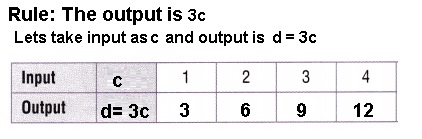Explanation:
Given the rule output is 3c means c is input lets take d as
output equal to d= 3c,
Used  the rule to make an input/output table as shown above ,
Included four input/output pairs in the table as
if c = 1 then d = 3  X 1  = 3,
if c = 2 then d = 3 X 2 = 6,
if c = 3 then d = 3 X 3 = 9,
if c = 4 then d = 3 X 4 = 12 as shown above in the table.

Question 3.
Rule: The output is d × 6.Explanation:
Given the rule output is d X 6 means d is input lets take e as
output equal to e= d X 6,
Used  the rule to make an input/output table as shown above ,
Included four input/output pairs in the table as
if d= 1 then e = 1 X 6  = 6,
if d = 2 then e = 2 X 6 = 12,
if d = 3 then e = 3 X 6 = 18,
if d = 4 then e = 4 X 6 = 24 as shown above in the table.

Question 4.
Rule: The output is 10 + b.Explanation:
Given the rule output is 10 + b means b is input lets take c as
output equal to c =10 + b,
Used  the rule to make an input/output table as shown above ,
Included four input/output pairs in the table as
if b = 1 then c = 10 + 1 = 11,
if b = 2 then c = 10 + 2 = 12,
if b = 3 then c = 10 + 3 = 13,
if b = 4 then c = 10 + 4 = 14 as shown above in the table.

Question 5.
Rule: The output is 8e.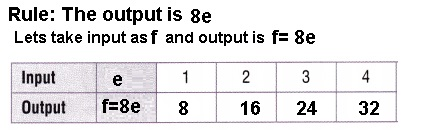Explanation:
Given the rule output is 8e means e is input lets take f as
output equal to f = 8e,
Used  the rule to make an input/output table as shown above,
Included four input/output pairs in the table as
if e = 1 then f = 8 X 1 = 8,
if e = 2 then f = 8 X 2 = 16,
if e = 3 then f = 8 X 3 = 24,
if e = 4 then f = 8 X 4 = 32 as shown above in the table.

Question 6.
Rule: The output is 12f.Explanation:
Given the rule output is 12f means f is input lets take g as
output equal to g = 12f,
Used  the rule to make an input/output table as shown above,
Included four input/output pairs in the table as
if g = 1 then g = 12 X 1 = 12,
if g = 2 then g = 12 X 2 = 24,
if g = 3 then g = 12 X 3 = 36,
if g = 4 then g = 12 X 4 = 48 as shown above in the table.

Question 7.
Rule: The output is 8 + g.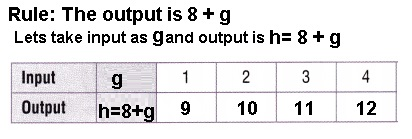Explanation:
Given the rule output is 8 + g means g is input lets take h as
output equal to h =8 + g,
Used  the rule to make an input/output table as shown above ,
Included four input/output pairs in the table as
if g = 1 then h = 8 + 1 = 9,
if g = 2 then h = 8 + 2 = 10,
if g = 3 then h = 8 + 3 = 11,
if g = 4 then h = 8 + 4 = 12 as shown above in the table.

Question 8.
Rule: The output is k × 2.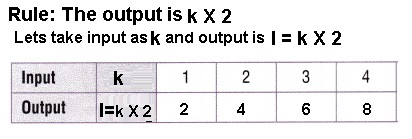Explanation:
Given the rule output is k x 2 means k is input lets take l as
output equal to l = k x 2,
Used  the rule to make an input/output table as shown above,
Included four input/output pairs in the table as
if k = 1 then l = 1 X 2 = 2,
if k = 2 then l = 2 X 2 = 4,
if k = 3 then l = 3 X 2 = 6,
if k = 4 then l = 4 X 2 = 8 as shown above in the table.

Problem Solving

Question 9.
Suki uses toothpicks to build a number of separate shapes for her math project.
She uses 1 toothpick for each side of a shape. Complete the input/output table to
show how many toothpicks Suki needs to make 6 hexagons.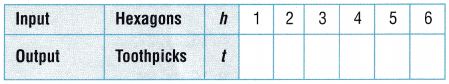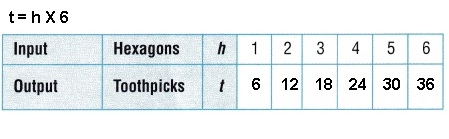number of toothpicks Suki needs to make 6 hexagons.
are 36 toothpicks,

Explanation:
Given Suki uses toothpicks to build a number of separate shapes for her math project.
She uses 1 toothpick for each side of a shape. Completed the input/output table to
show how many toothpicks Suki needs to make 6 hexagons.
as we know hexagon means six sides so rule is t = h x 6(sides)  means t  is
toothpicks needed as input and h is hexagons so output equal to t = h x 6,
Used  the rule to make an input/output table as shown above,
if h= 1 then t = 1 X 6 = 6,
if h = 2 then t = 2 X 6 = 12,
if h = 3 then t = 3 X 6 = 18,
if h = 4 then t = 4 X 6 = 24,
if h = 5 then t = 5 X 6 = 30,
if h = 6 then t = 6 X 6 = 36 as shown above in the table,
therefore number of toothpicks Suki needs to make 6 hexagons.
are 36 toothpicks.

Lesson Check

Question 10.
If c is cups of sugar and b is number of batches of cookies,
which rule matches the information in the table?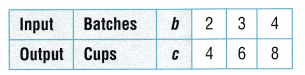(A) The output is b × 2.
(B) The output is c × 2.
(C) The output is b + 2.
(D) The output is c + 2.
The rule that matches the information in the table is
(A) The output is b × 2,

Explanation:
Given if c is cups of sugar and b is number of batches of cookies
by seeing the information in the table input b = 2,3,4 and output
c is 4,6,8 if we check (A) b x 2 means
if b = 2 c is 2 X 2 = 4,
if b= 3, c is 3 X 2 = 6,
if b = 4, c is 4 X 2 = 8,
if we check (B) c X 2 output multiplied again by 2 means it will not match,
if we check (C) b + 2 means
if b = 2, c= 2 + 2 = 4,
if b = 3, c= 3 + 2 = 5,
if b = 4, c = 4 + 2 = 6, which is not matching and
if we check (D) c + 2 means output again added by 2 means which will not match,
therefore the rule that matches the information in the table is
(A) The output is b × 2.

Question 11.
Tia hangs chili-shaped party lights in her back yard.
The rule c — 14/gives the number of chili lights, c,
there are for each foot,/ of wire. How many lights are on 8 feet of wire?
(A) 126
(B) 112
(C) 98
(D) 96
Number of lights on 8 feet of wire is 112, matches with (B),

Explanation:
Given Tia hangs chili-shaped party lights in her back yard.
The rule c — 14/gives the number of chili lights, c,
there are for each foot,/ of wire, number of lights are on
8 feet of wire are 14 X 8 = 112 which matches with (B).

Question 12.
Harlan uses the pattern rule l = d + 2 to represent the number of laps
she swims each day for a week. Which is the number of laps she swims on the sixth day?(A) 6
(B) 7
(C) 8
(D) 9
8 laps which matches with (C),

Explanation:
Given Harlan uses the pattern rule l = d + 2 to represent the number of laps
she swims each day for a week.
So the number of laps she swims on the sixth day is l = 6 + 2 = 8 laps,
which matches with (C).

Question 13.
Erik uses the equation e = 25b to determine how much he earns for
selling hand-carved bowls at the craft fair. If b represents the number of
bowls sold and e represents his earnings, how much will Erik earn if he sells 9 bowls?(A) $225 (B)$100
(C) $150 (D)$45
Erik earns $225 if he sells 9 bowls which matches with (A), Explanation: Given Erik uses the equation e = 25b to determine how much Erik earns for selling hand-carved bowls at the craft fair. If b represents the number of bowls sold and e represents his earnings, Erik earns if he sells 9 bowls is 25 X 9 =$225 which matches with (A).

Question 14.
Multi-Step Gina paints beach scenes on notecards.
She sells packages of 8 notecards for $8 for one package,$16 for 2 packages, and $24 for 3 packages. Which equation is the rule for the pattern if n is the number of packages and d is the selling price in dollars? (A) n = 8d (B) d = 8 + n (C) n = 8 + d (D) d = 8n Answer: The rule for the pattern is d = 8n matches with (D), Explanation: Given Gina paints beach scenes on notecards. She sells packages of 8 notecards for$8 for one package,
$16 for 2 packages, and$24 for 3 packages.
Now the rule for the pattern if n is the number of packages and
d is the selling price in dollars
if (A) matches means if d = $1, n = 8 packages if d= 2, n = 16 and if d =3, n= 24 packages which will not match, if (B) matches means if n = 1, d = 8 + 1 =$9 ,
if n = 2, d = 8 + 2 = $10 which will not at match, If (C) if d$1, n = 9 packages
if d= 2, n = 10 and if d =3, n= 11 packages which will not match,
if (D) matches means if n = 1, d = $8, if n = 2 means d =$16 and if n = 3 means d = \$24 which matches,
so The rule for the pattern is d = 8n matches with (D).

Question 15.
Multi-Step At his job at the deli, Sam makes 9 sandwiches and 6 salads each hour.
Which of the following patterns can you use to find the number of sandwiches he makes in 4 hours?
(A) 9, 18, 27, 36, ………
(B) 6, 12, 18, 24,……..
(C) 9, 15, 21, 27, ………
(D) 8, 16, 24, 28, ……….
Pattern (A) 9, 18, 27, 36,…………

Explanation:
Given at his job at the deli, Sam makes 9 sandwiches and 6 salads each hour.
We see which of the following patterns can we use to find the
number of sandwiches he makes in 4 hours are
1. if (A) means 1 hour 9 sandwiches , 2 hour= 2 x 9 sandwiches = 18,
3 hour = 3 x 9 sandwiches = 27 and 4th hour = 4 x 9 sandwiches = 36
which exactly matches,
Now (B) means 1 hour it is showing 6 but each hour it is 9 not 6 so does not
at all match,
If (C) 1 hour 9 sandwiches , 2 hour= 2 x 9 sandwiches = 18 ≠  15,
3 hour = 3 x 9 sandwiches = 27 ≠  21 and 4th hour = 4 x 9 sandwiches = 36 ≠ 27
which means it will not match,
Now if (D) means 1 hour it is showing 8 but each hour it is 9 not 8 so does not
at all match,
therefore Pattern (A) 9, 18, 27, 36,………… exactly matches.

Scroll to Top